#Real-Field Model: II

Consider a two-phase oil-water problem. Solve the two-phase pressure equation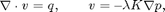where v is the Darcy velocity (total velocity) and lambda is the mobility, which depends on the water saturation S.

The saturation equation (conservation of the water phase) is given as: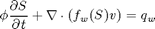where phi is the rock porosity, f is the Buckley-Leverett fractional flow function, and q_w is the water source term.

## Read and process the model

We start by reading the model from a file in the Eclipse formate (GRDECL). In Real-Field Model: I, we examined the model in more detail, and showed that the grid has two components, of which we will only use the first one.

```grdecl = readGRDECL(fullfile(ROOTDIR, 'examples', 'grids', 'GSmodel.grdecl'));
G = processGRDECL(grdecl, 'Verbose', false); clear grdecl;
G = computeGeometry(G(1), 'Verbose', false);
```

## Set rock and fluid data

The permeability is lognormal and isotropic within nine distinct layers and is generated using our simplified 'geostatistics' function and then transformed to lay in the interval 200-2000 mD. For the permeability-porosity relationship we use the simple relationship that phi~0.25*K^0.11, porosities in the interval 0.25-0.32. For the two-phase fluid model, we use values:

• densities: [rho_w, rho_o] = [1000 700] kg/m^3
• viscosities: [mu_w, mu_o] = [1 5] cP.
```gravity off
K          = logNormLayers(G.cartDims, rand(9,1), 'sigma', 2);
K          = K(G.cells.indexMap);
K          = 200 + (K-min(K))/(max(K)-min(K))*1800;
rock.perm  = K*milli*darcy;
rock.poro  = 0.25*(K/200).^0.1; clear K;
fluid      = initSimpleFluid('mu', [1 5]);

clf,
plotCellData(G,log10(rock.perm),'EdgeColor','k');
axis off, view(15,60), h=colorbar('horiz');
cs = [200 400 700 1000 1500 2000];
caxis(log10([min(cs) max(cs)]*milli*darcy));
set(h, 'XTick', log10(cs*milli*darcy), 'XTickLabel', num2str(round(cs)'));
zoom(2.5), title('Log_{10} of x-permeability [mD]');
```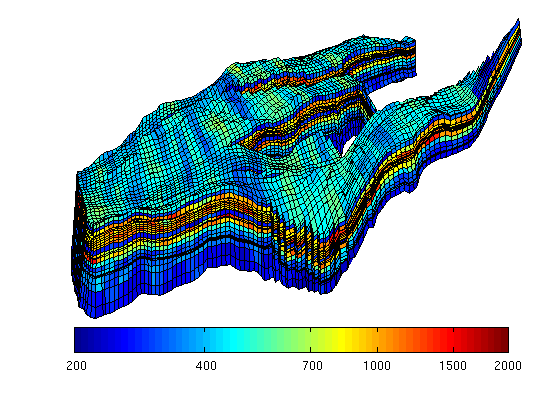## Initialize and construct linear system

Initialize solution structure with reservoir pressure equal 350 bar. Assemble linear hybrid system from input grid and rock properties.

## Introduce wells

The reservoir is produced using a set production wells controlled by bottom-hole pressure and rate-controlled injectors. Wells are described using a Peacemann model, giving an extra set of equations that need to be assembled. For simplicity, all wells are assumed to be vertical and are assigned using the logical (i,j) subindex.

```% Set vertical injectors, completed in the lowest 12 layers.
nz = G.cartDims(3);
I = [ 9, 26,  8, 25, 35, 10];
J = [14, 14, 35, 35, 68, 75];
R = [ 4,  4,  4,  4,  4,  4]*1000*meter^3/day;
nIW = 1:numel(I); W = [];
for i = 1 : numel(I),
W = verticalWell(W, G, rock, I(i), J(i), nz-11:nz, 'Type', 'rate', ...
'Val', R(i), 'Radius', 0.1, 'Comp_i', [1,0,0], ...
'name', ['I\$_{', int2str(i), '}\$']);
end

% Set vertical producers, completed in the upper 14 layers
I = [17, 12, 25, 35, 15];
J = [23, 51, 51, 95, 94];
nPW = (1:numel(I))+max(nIW);
for i = 1 : numel(I),
W = verticalWell(W, G, rock, I(i), J(i), 1:14, 'Type', 'bhp', ...
'name', ['P\$_{', int2str(i), '}\$']);
end

% Plot grid outline and the wells
clf
subplot('position',[0.02 0.02 0.96 0.96]);
plotGrid(G,'FaceColor','none','EdgeAlpha',0.1);
axis tight off, zoom(1.1), view(-5,58)
plotWell(G,W,'height',200);
plotGrid(G, vertcat(W(nIW).cells), 'FaceColor', 'b');
plotGrid(G, vertcat(W(nPW).cells), 'FaceColor', 'r');
```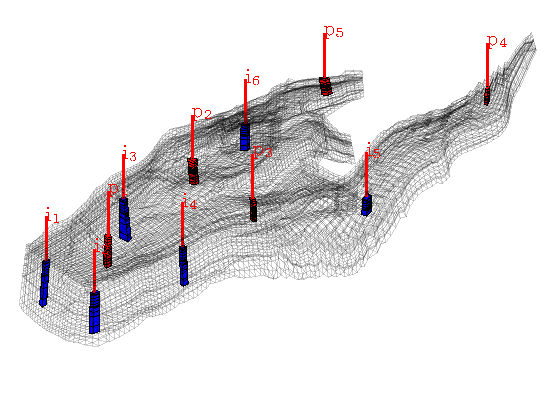## Initialize and construct the linear system

Initialize solution structures and assemble linear hybrid system from input grid, rock properties, and well structure.

```S    = assembleMimeticSystem(G, rock, 'Verbose', true);
W    = assembleWellSystem(G, W);
rSol = initResSol(G, 350*barsa, 0.0);
wSol = initWellSol(W, 300*barsa());
```
```Using inner product: 'ip_simple'.
Computing component matrices C and D ... Elapsed time is 0.018762 seconds.
Computing cell inner products ... Elapsed time is 6.298584 seconds.
Assembling global inner product matrix ... Elapsed time is 0.097781 seconds.
```

## Solve initial pressure

Solve linear system construced from S and W to obtain solution for flow and pressure in the reservoir and the wells.

```[rSol, wSol] = solveIncompFlow(rSol, wSol, G, S, fluid, 'wells', W);
clf
plotCellData(G, convertTo(rSol.cellPressure, barsa), 'EdgeColor','k');
title('Initial pressure'), colorbar('horiz')
plotWell(G,W,'height',200,'color','w');
axis tight off; view(20,80);
zoom(2)
```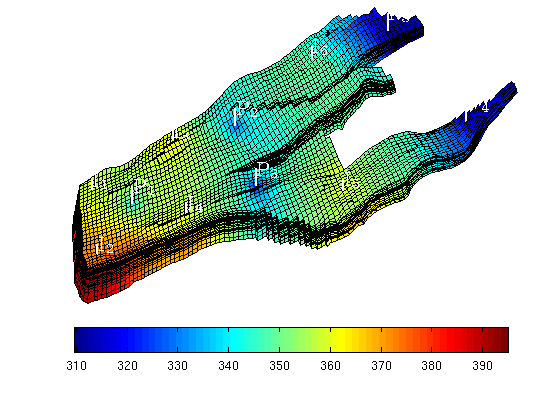## Main loop

In the main loop, we alternate between solving the transport and the flow equations. The transport equation is solved using the standard implicit single-point upwind scheme with a simple Newton-Raphson nonlinear solver.

```T      = 30*year();
dT     = T/12;
dTplot = 5*year();
pv     = poreVolume(G,rock);

% Prepare plotting of saturations
clf
plotGrid(G,'FaceColor','none','EdgeAlpha',0.1);
plotWell(G,W,'height',200,'color','c');
axis off, view(30,50), colormap(flipud(jet))
colorbar('horiz'); hs = []; ha=[]; zoom(2.5)

% Start the main loop
t  = 0;  plotNo = 1;
while t < T,
```
```   rSol = implicitTransport(rSol, wSol, G, dT, rock, fluid, 'wells', W);

% Check for inconsistent saturations
assert(max(rSol.s) < 1+eps && min(rSol.s) > -eps);

% Update solution of pressure equation.
[rSol, wSol] = solveIncompFlow(rSol, wSol, G, S, fluid, 'wells', W);

% Increase time and continue if we do not want to plot saturations
t = t + dT;
if ( t < plotNo*dTplot && t ```

Plot saturation

```   delete([hs, ha])
hs = plotCellData(G, rSol.s,find(rSol.s>0.01));
ha = annotation('textbox',[0.6 0.2 0.5 0.1], 'LineStyle','none', ...
'String', ['Water saturation at ',num2str(convertTo(t,year)),' years']);
view(30, 50+7*(plotNo-1)), drawnow
plotNo = plotNo+1;
```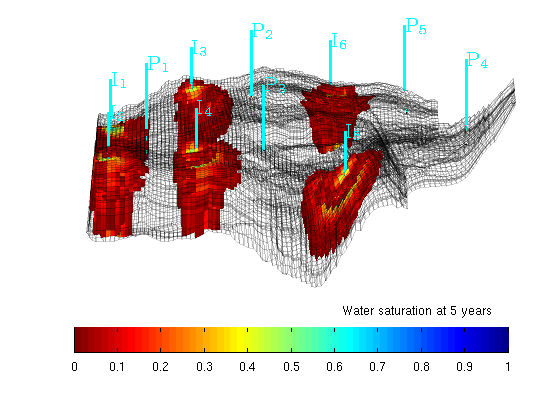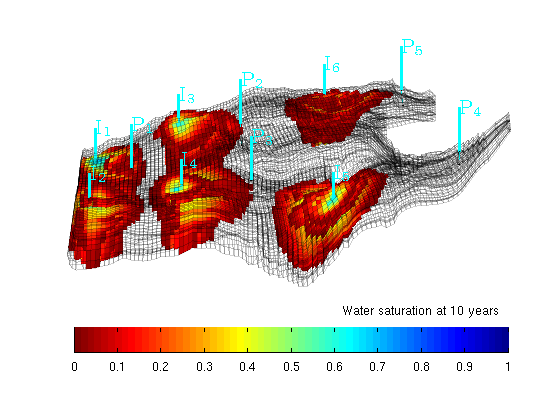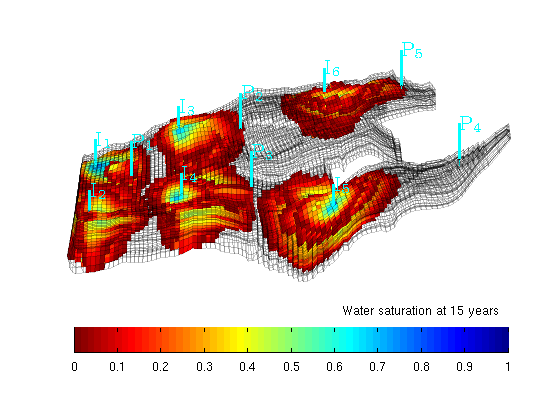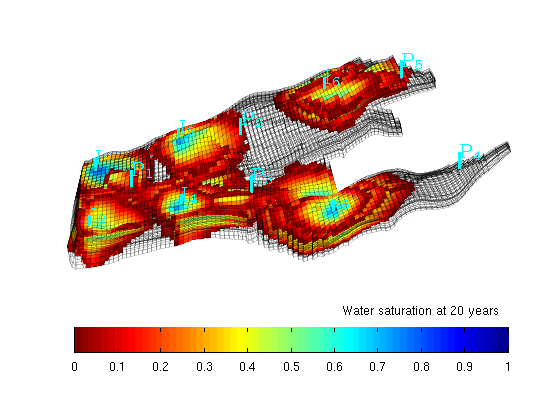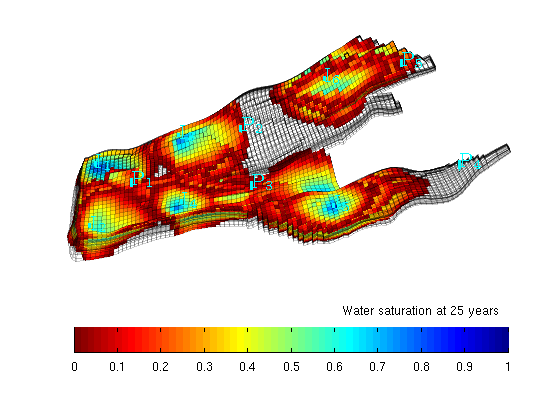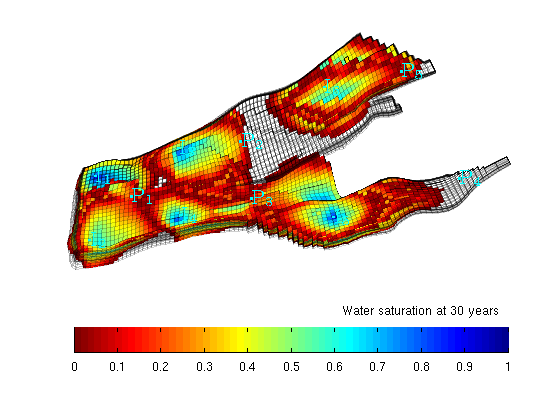```end
```

Published March 28, 2009